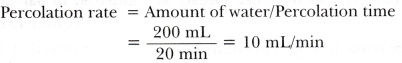# Rahul conducted an experiment to calculate the rate of percolation. He observed that it took 20 minutes for 200 mL of water to percolate through the soil sample. Calculate the rate of percolation

Rahul conducted an experiment to calculate the rate of percolation. He observed that it took 20 minutes for 200 mL of water to percolate through the soil sample. Calculate the rate of percolation.